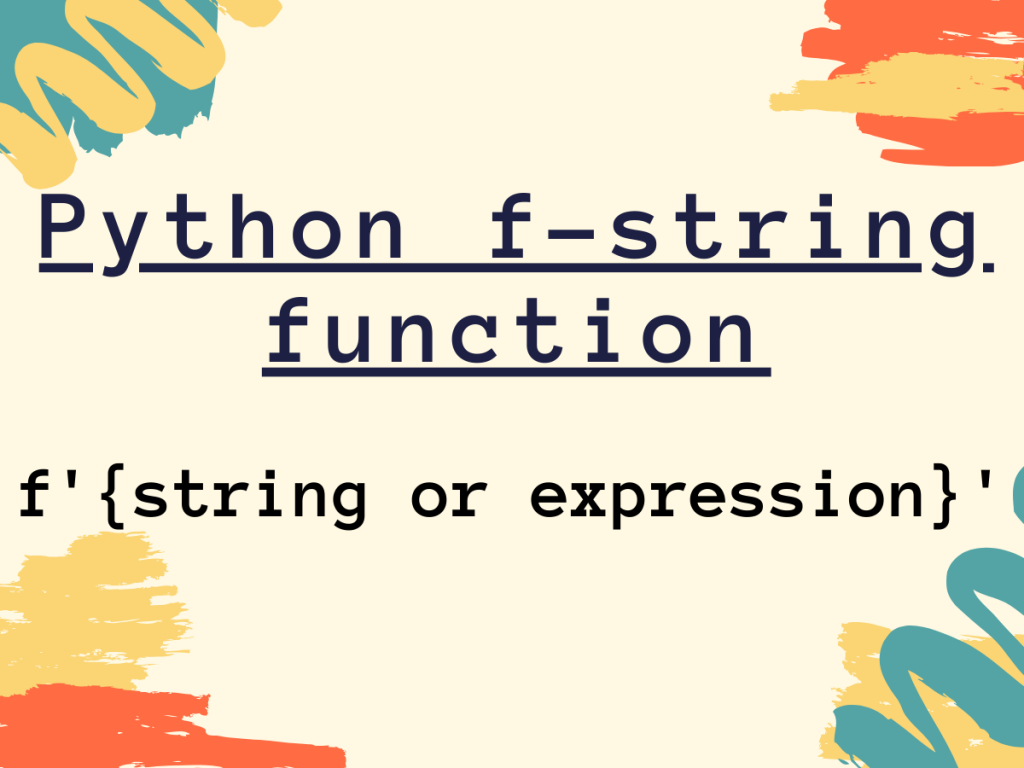# Understanding Python f-stringString formatting in Python can be achieved using f-string. Thus, in this article, we will be focusing on the implementation of Python f-string.

## Necessity of f-string in Python

Python f-string basically serves the purpose of string formatting. Before the emergence of “f-strings”, we had the following ways to format strings in Python:

1. Python ‘%’ operator

Disadvantage: Python `% operator` cannot be used with Objects and attributes.

2. Python format() function —

Disadvantage: The `string.format() function` could overcome the drawback of ‘%’ operator, but it proved out to be a verbose way of formatting.

Thus, Python f-string came into existence wherein the strings can be interpolated and formatted with much simpler and minimal syntax. The strings are formatted at runtime by the Python interpreter.

## Working of Python f-string with examples

The f-string also known as formatted strings serves the purpose of Literal String Interpolation i.e. injection of strings and formatting of the particular string.

Syntax:

```f'{string}'
```

Example: f-string with string as an iterable

```str1 = 'Python'

str4 = 'JournalDev'

res = f'{str4} provides tutorials on {str1}'

print(res)
```

As clearly seen above, f-string is used to inject or interpolate the input string str1 and str4 between the string statement.

Output:

```JournalDev provides tutorials on Python
```

### Python f-string with raw strings

Python raw strings basically treat the special characters considered as ‘escape sequences’ as literal characters. It is used when we want the escape sequences i.e. ‘\n’ or backslash(\) as literal sequences of characters.

Syntax: Python raw strings

```r'string'
```

Python f-strings can work well simultaneously with the raw strings.

Syntax: f-string along with raw strings

```fr'string or {string}'
```

Example:

```str1 = 'Python'

str4 = 'JournalDev'

res = fr'{str4} \n and AskPython provides tutorials on {str1}'

print(res)

```

In the above example, ‘\n’ is treated as a literal character.

Output:

```JournalDev \n and AskPython provides tutorials on Python
```

### Calling functions with f-string

Python f-strings enables us to call functions within it. Thus, optimizing the code to an extent. The same way can be used for creating lambda functions within f-string bracets.

Syntax:

```f'{func()}'
```

Example:

```def mult(a,b):
res = a*b
return res

mult_res = f'{mult(10,20)}'
print(mult_res)
```

Output:

```200
```

### Python f-string with blank/white-spaces

Python f-strings can also work with blank or white-spaces. It ignores the trailing and the leading white-spaces and the spaces between the literal string are unaltered and preserved.

Example:

```mult_res = f'  Multiplication: { 10 * 10 }  '
print(mult_res)
```

Output:

```  Multiplication: 100
```

### Python f-string with expressions

Python f-string can work with expressions. Thus, basic manipulations can be performed directly within f-string.

Syntax:

```f'{expression'}
```

Example:

```x = 10
y = 5
print(f'Result: {x/y} ')

```

Output:

```Result: 2.0
```

### f-string with a Python dictionary

As we all know, Python dictionary data structure works with key-value pairs. Python f-string can also be framed along with the dictionaries.

Syntax:

```f"{dict['key']}"
```

Example:

```info = {'age':'21', 'city':'Pune'}

print(f"{info['city']}")
```

Output:

```Pune
```

## Conclusion

Thus, in this article, we have understood the necessity and working of f-string with various iterables and expressions.